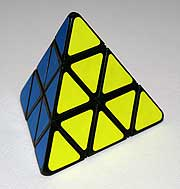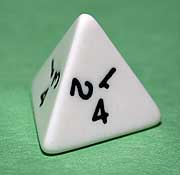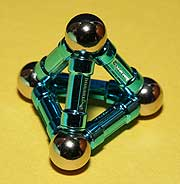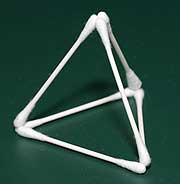JBQ's spot on the Wild Wild Web The musings of a French mathematician living in the heart of the American technology industry

The regular tetrahedron
The regular tetrahedron is the most basic of all polyhedra. It has 4 faces, which are all equilateral triangles. It has 6 edges and 4 vertices. As a general form, the tetrahedron is the ony polyhedron with 4 faces, it is the only polyhedron with 6 edges, and it is the only polyhedron with 4 vertices, and no polyhedron can be built with fewer faces, with fewer edges, or withfewer vertices; that means that any effort to build any nomenclature of polyhedra starting from the smallest number of faces, of edges or of vertices always starts with a tetrahedron. Following that natural order, the regular tetrahedron is therefore the first polyhedron that I write about.

The regular tetrahedron is part of the family of 5 platonic solids, which are the most regular polyhedra. It is also part of the family of 8 convexdeltahedra (because it is convex and all its faces are equilateral triangles), and it is the first member of the infinite family of pyramids (one of the vertices shares identical edges with all the other vertices, which are arranged as a regular polygon).

The tetrahedron's simplicity gives it a few other unusual or unique properties. It is the only convex polyhedron in which the straight line between any two vertices is always an edge (mathematically, that means thatthe tetrahedron has no polyhedron diagonals, just like a triangle in two dimensions). If you join the centers of the faces that share a common edge (mathematically, that's called creating the dual polyhedron), you get another tetrahedron, and the faces of the dual have the same shape as the faces of the original; the tetrahedron is therefore called self-dual.

The regular tetrahedron defines two sets of symmetry axes: one set of 4 3-fold axes that join each vertex to its opposite face, and one set of 3 2-fold axis that join the middle of opposite edges.

Tetrahedra occur in nature, and especially in chemistry: the 4 hydrogen atoms of a methane molecule are exactly located at the vertices of atetrahedron. The same is true for the hydrogen atoms in the ammonium ion, and for the atoms surrounding the central carbon in tetrafluoromethane, tetrachloromethane, tetrabromomethane and tetraiodomethane. The same molecular geometry occurs in perchlorate, sulfate and phosphate ions. Finally, the atoms in diamond and in silicon crystals follow a tetrahedral geometry.

The regular tetrahedron is the shape that is used to build 4-sided dice.

The regular tetrahedron can be built with various construction toys, like Zome, Magnetix (see article), Googolplex and Polymorf.

The following table lists the various dimensions in a regular tetrahedron, assuming that the edge length is 1:

 single edge length 1 total edge length 6 face inradius 0.288 face circumradius 0.577 polyhedron inradius 0.204 polyhedron midradius 0.354 polyhedron circumradius 0.612 single face area 0.433 total face area 1.732 volume 0.118

The following table is the reciprocal of the previous one, it lists the edge length that yields a value of 1 fo the different measurements in a regular tetrahedron:

 1 single edge length 0.167 total edge length 3.464 face inradius 1.732 face circumradius 4.899 polyhedron inradius 2.828 polyhedron midradius 1.633 polyhedron circumradius 1.52 single face area 0.76 total face area 2.04 volume

The following table lists various angles in a regular tetrahedron:

 angle between vertices (from center) 109.5 degrees angle between edges 60 degrees angle between faces 70.5 degrees